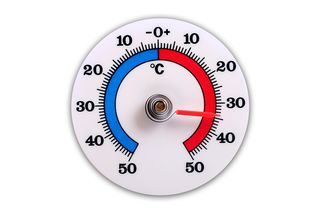# Celsius: Facts, Formulas & HistoryOn the Celsius temperature scale, 0 degrees is the freezing point of water.

Celsius is a measurement of temperature in which 0 degrees represents the freezing point of water, and 100 degrees represents water’s boiling point at the standard atmosphere, which is the mean barometric pressure at the mean sea level.

This temperature scale was developed by Swedish astronomer Andres Celsius in 1742. Celsius is credited with being the first astronomer to analyze the changes in the Earth’s magnetic field, and he developed measuring tools to assess the brightness of stars.

Celsius performed diligent experiments to develop his scale, although he originally had 0 as the boiling point and 100 as the freezing point. In his paper, "Observations of two persistent degrees on a thermometer," he documented experiments to determine that water’s freezing point is independent of latitude or atmospheric pressure. On the other hand, he found that latitude and atmospheric pressure do affect the boiling point of water.

Celsius was not the only scientist coming up with temperature scales at the time. Another scale, developed by Daniel Gabriel Fahrenheit, was the standard for quite a while. Fahrenheit used his scale on the alcohol and mercury thermometers he invented in the early 1700s. However, many considered Celsius’ scientific methods to be more precise and meticulously executed than those of Fahrenheit.

French physicist Jean-Pierre Christin developed a scale similar to Celsius' several years later, but he had 0 degrees representing the freezing point and 100 degrees the point where water boils.

Celsius did not live to see his scale develop into a standard, as he died in 1744. His death prompted a move to officially invert the scale, and by 1747 the scale was already changed on some thermometers.

For many years, the scale was referred to as centigrade, but the name changed in 1948. The Conference General des Poids et Measures decided to standardize several units of measurement, including the temperature scale. Since "grade" was already in use as a unit of measurement, Celsius was chosen to avoid confusion.

## Who uses Celsius?

Celsius is used in most scientific applications and it is the most widely used temperature scale throughout the world. Celsius makes sense for many scientific applications, because a Celsius degree is the same size as degree Kelvin, another scale favored by scientists, making it easier to work with the units and calculations that use Kelvin.

While the United States and a few territories still uses the Fahrenheit scale, nearly all countries switched over to Celsius in the 1970s as a move to standardize on metric measurement system. Canadian media will often report temperatures in both Fahrenheit and Celsius, particularly in areas close to the border with the United States.

While non-scientists in the United States still favor the Fahrenheit scale, American scientists use the Celsius scale, as they need to share their data with scientists around the world.

## Conversion formulas

• Celsius to Fahrenheit: Multiply by 9, divide by 5, then add 32
• Fahrenheit to Celsius: Subtract 32, then multiply by 5, then divide by 9
• Celsius to Kelvin: Subtract 273
• Kelvin to Celsius: Add 273